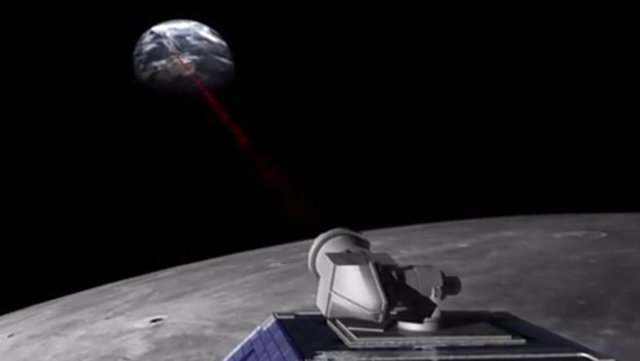# Einstein’s Relativity prevails over the doubts of quantum physicistsJuly 14 () –

One of the most basic assumptions in fundamental physics is that the different properties of mass (weight, inertia, and gravitation) they always remain equal to each other.

Without this equivalence, Einstein’s theory of relativity would be contradicted and our current physics textbooks would have to be rewritten. Although all measures to date confirm the principle of equivalence, quantum theory postulates that there should be a violation.

This inconsistency between Einstein’s gravitational theory and modern quantum theory is the reason why more and more precise proofs of the equivalence principle are particularly important.

A team from the Center for Applied Space Technology and Microgravity (ZARM) at the University of Bremen, in collaboration with the Institute for Geodesy (IfE) at the Leibniz University of Hannover, has succeeded in demonstrating with 100 times greater precision than passive gravitational mass and the active gravitational mass are always equivalent, regardless of the particular composition of the respective masses.

The research was carried out within the framework of the “QuantumFrontiers” Cluster of Excellence. The team is now publishing their findings as a featured article in Physical Review Letters.

Inertial mass resists acceleration. For example, it causes you to be pushed back in your seat when the car starts. Passive gravitational mass reacts with gravity and results in our weight on Earth. Active gravitational mass refers to the gravitational force exerted by an object, or more precisely, the size of its gravitational field.

The equivalence of these properties is fundamental to general relativity. Therefore, both the equivalence of inertial and passive gravitational mass and the equivalence of passive and active gravitational mass are being tested with increasing precision.

If we assume that the passive and active gravitational mass are not equal, that their relationship depends on the material, then objects made of different materials with a different center of mass would accelerate. Since the moon consists of an aluminum shell and an iron core, with centers of mass displaced relative to each other, the moon should be accelerating. This hypothetical change in speed could be measured with high precision, through “Lunar Laser Ranging”.

This involves pointing lasers from Earth at reflectors on the Moon placed there by the Apollo missions and the Soviet Luna program. Since then, the round-trip travel times of the laser beams have been recorded. The research team analyzed “Lunar Laser Range” data collected over a 50-year period, from 1970 to 2022, and investigated such mass difference effects.

Since no effect was found, this means that the passive and active gravitational masses are equal to approximately 14 decimal places. This estimate is a hundred times more accurate than the best previous study, which dates back to 1986.

One of only four centers worldwide that analyze laser distance measurements to the moon, the LUH Institute of Geodesy has unique expertise in evaluating the data, particularly for testing general relativity. In the current study, the institute analyzed lunar laser ranging measurements, including error analysis and interpretation of the results.